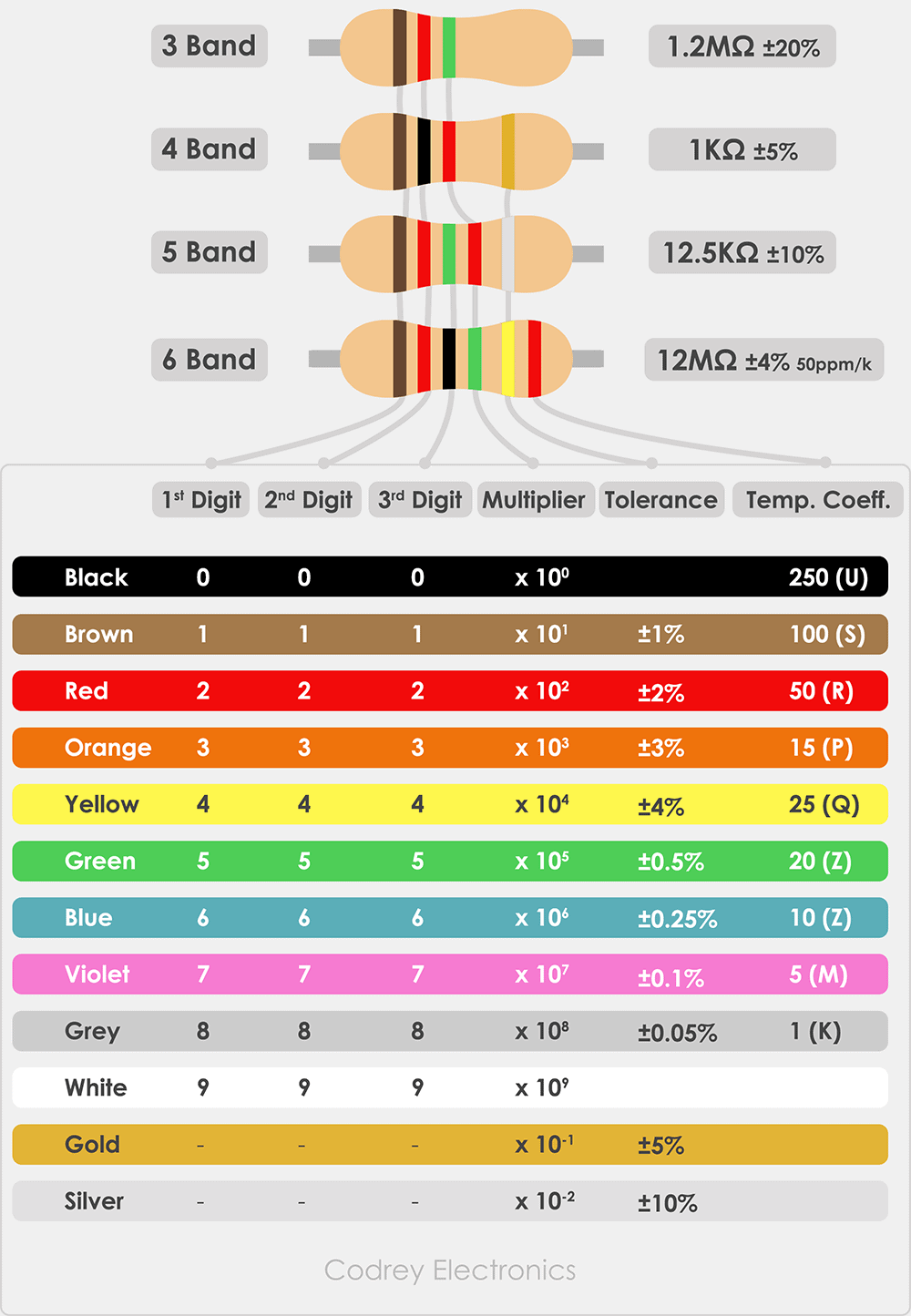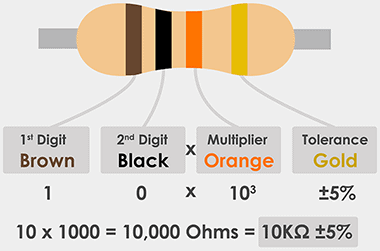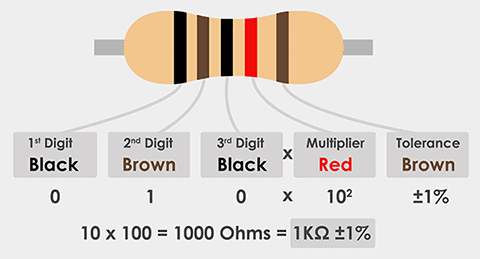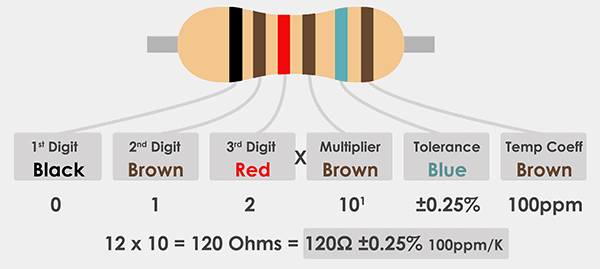# Resistor Color Code Calculator

The resistor color code was developed during the year 1920. The color bands are printed on the body of tiny resistor components. Generally, for color code, we can use this resistor mnemonic called BBROY Great Britain Very Good Wife. Whereas, the beginning letter indicates a unique color.

This color-coding shortcut has an acronym for how to identify a resistor value.

Resistor Color Code Representation
Color Code Resistor Acronym Band Color
0 B
Black
1 B
Brown
2 R
Red
3 O
Orange
4 Y
Yellow
5 G
Green
6 B
Blue
7 V
Violet
8 G
Grey
9 W
White

Resistors use the BS1852 (British standard) coding standard for value representation. It uses letter 'R' for ohms, 'K' for Kilo ohms, and 'M' for Megaohms. For example, a 4.7K ohm resistor is shown as 4K7.

## Resistor Color Bands

The Carbon composition resistors have 3 to 6 resistor color bands. The 3-band resistor has three colors with multiplier and no tolerance.

The three bands can be selected to know the resistor value. Whereas, the 4 band, 5 band, and 6 band resistors have an extra band known as tolerance.

The color code chart shows the 3 bands, 4 band, 5 band, and 6 band resistor strips.

3 Band Resistor 4 Band Resistor 5 Band Resistor 6 Band Resistor
1st band First Digit First Digit First Digit First Digit
2nd band Second Digit Second Digit Second Digit Second Digit
3rd band Multiplier Value Multiplier Value Third Digit Third Digit
4th band Tolerance Value Multiplier Value Multiplier Value
5th band Tolerance Value Tolerance Value
6th band Temperature Coefficient

To know, how to find color code, each color indicates a number starting from 0 to 9. This number can be used as the first significant digit and second significant digit for 3 band and 4 band. For 5 band and 6 band resistors, the first 3 digits indicate significant numbers.

The multiplier value is multiplied with the significant digit number (one, two, or three digits) to get the desired resistance value. In addition to this the 4 band, 5 band, and 6 band resistors have tolerance value ranging from ±0.10 to ±10.

The 6-band resistor has a special property of temperature coefficient of resistance represented in ppm/Kelvin. The higher ppm value indicates that a resistor can withstand the higher or lower temperature. The variation of resistance is constant to temperature.

## Resistor Color Code Chart – 3 band 4 band 5 band and 6 band

To understand how to read the resistor color code for 3 /4/5/6 band resistors you can use this color chart.From the above chart, each color band on the resistor represents a number. For example, to calculate 1.2MΩ, the resistor shows Brown Red Green colors (read from left side to right). Now place the first two bands as the numeric value and third band as a multiplier (105).

## Deviations in Resistor Color Coding

Reliability

To meet the military specification resistors often manufactured with reliability band. This band is not found in commercial electronics. Typically, a 4-band resistor comes with a reliability band.

Zero Ohm resistor

This resistor comes with a single black band used to connect the traces on a Printed Circuit Board (PCB). It is used as an interconnection between two joints.

Resistor bands with Gold and Silver

The gold and silver bands are prone to misconceptions of true colors in a resistor. Hence, they are replaced with grey and yellow color bands.

## How to Use the Resistor Color Code Calculator

The resistor calculator tool calculates the color code for 3band, 4 band, 5 band, and 6 band resistors typically in the range of ohms, Kilo Ohms, and Mega Ohms.

The resistance calculator has 1 to 6 band colors with Multiplier (Mul) and tolerance (Tol) and PPM/Kelvin. You must select the right color corresponding to each column. The tolerance value tells the accuracy at which the resistor is manufactured. Normally, for gold, it is 5% and for silver, it is 10%.

This will display the true resistance value with tolerance and temperature coefficient of resistance.

## Resistor Color Code Examples

To explore more, let’s discuss a few examples of resistor color code for 4, 5, and 6 band resistors.

4 band Color Code

For example, 4-band resistor has color Brown Black Orange Gold. What's the resistor value?By using the color chart, write the values as, 1/0/103 = 10 * 103 = 10K ohm/10K. The tolerance is ±5% for Gold. So, the value ranges from 9.5KΩ to 10.5KΩ.

5 Band Color Code

Another example (From chart): 5-band resistor has color Black – Brown Black Red Brown.Write the values as, 0/1//0/102 = 10 * 100 = 1K ohm/1K. The tolerance is ±1% for Brown. Hence, the resistor value is 900Ω to 1.01KΩ

6 Band Color Code

A 6-band resistor has Black Brown Red Brown Blue BrownUsing the chart, the values are 0/1/2/101 = 120 0hm/120R. The tolerance is ±0.25% for Blue and the temperature coefficient of resistance is 100ppm. So, the resistance becomes 119.7Ω -120.3Ω

## Standard Resistor Values

Electronic color coding is standardized by the Electronic Industries Association (EIA) and later by the Radio Manufacturers Association (RMA) as standard color marking for resistors.

This marking code changes from one decade to another decade. This is known as the EIA color code. For each tolerance band, the EIA allocates E-series (E3, E6, E12, E24 and E96) to name the resistor values.

The standard decade resistors (also known as preferred values) are shown in the below table. The resistance calculation is starting from 1 ohm with tolerance range (36%, 10%, 5% and 1%).

E3 Series - Resistance with ±36% Tolerance (Value in Ohms)
1.0 2.2 4.7
E6 Series - Resistance with ±20% Tolerance (Value in Ohms)
1.0 1.5 2.2 3.3 4.7 6.8
E12 Series - Resistance with ±10% Tolerance (Value in Ohms)
1.0 1.2 1.5 1.8 2.2 2.7 3.3 3.9 4.7 5.6
E24 Series - Resistance with ±5% Tolerance (Value in Ohms)
1.0 1.1 1.2 1.3 1.5 1.6 1.8 2.0 2.2 2.4
2.7 2.9 3.0 3.3 3.6 4.3 4.7 5.1 5.6 6.2
6.8 7.2 8.2 9.1
E96 Series - Resistance with ±1% Tolerance (Value in Ohms)
1 1.02 1.05 1.07 1.10 1.13 1.15 1.18 1.21 1.24
1.27 1.30 1.33 1.37 1.40 1.43 1.47 1.50 1.54 1.58
1.62 1.65 1.69 1.74 1.78 1.82 1.87 1.91 1.96 2.00
2.05 2.10 2.15 2.21 2.26 2.32 2.37 2.43 2.49 2.55
2.61 2.67 2.74 2.80 2.87 2.94 3.01 3.09 3.16 3.24
3.32 3.40 3.48 3.57 3.65 3.74 3.83 3.92 4.02 4.12
4.22 4.32 4.42 4.53 4.64 4.75 4.87 4.99 5.11 5.23
5.36 5.49 5.62 5.76 5.90 6.04 6.19 6.34 6.49 6.65
6.81 6.98 7.15 7.32 7.50 7.68 7.87 8.06 8.25 8.45
8.66 8.87 9.09 9.31 9.53 9.76

To find the resistor values from E-series, take the preferred resistor with tolerance. We will get resistances by multiplying value with the multiplier constant. For example, E6 series resistor (1 ohm) with tolerance ±20, the set of resistances is 1, 20, 400, 8K, 160K.

## Conclusion

Carbon strip resistors do not have their resistance value and tolerance printed on their body due to their size. Hence the resistor color code chart and calculator help to find out the resistor value without using a digital multimeter.

Here are some points to remember on resistor color coding.

1. Resistor color code saying the Fifth band is black, which type of resistor?

For a wire wound resistor, the 5th band is black and for a fusible resistor, the 5th band is white color. If the only single band (black color) in the middle it is a zero-ohm resistor.

2. From which side should I read the resistor?

Read from the left side to right. The idea is, the gold or silver bands (for tolerance) are present on the right side. If the gold, silver bands are not present, then the band that is close to the lead will be the first band.

3. Which type of E-Series is mostly used?

In most of the circuits, the preferred series are E6, E12, and E24. E96 series are high in cost as their tolerance is less.